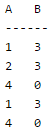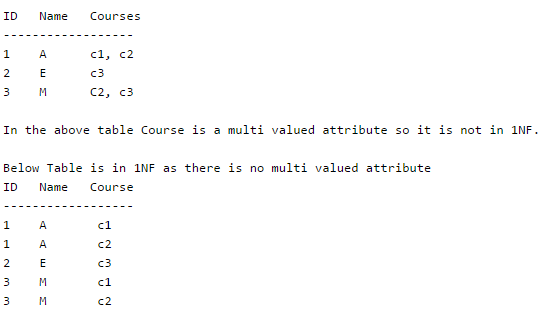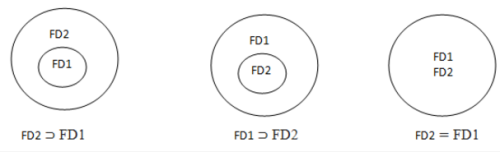Courses

# Normal Forms Computer Science Engineering (CSE) Notes | EduRev

## Computer Science Engineering (CSE) : Normal Forms Computer Science Engineering (CSE) Notes | EduRev

The document Normal Forms Computer Science Engineering (CSE) Notes | EduRev is a part of the Computer Science Engineering (CSE) Course GATE Computer Science Engineering(CSE) 2022 Mock Test Series.
All you need of Computer Science Engineering (CSE) at this link: Computer Science Engineering (CSE)

Introduction

Database normalization is the process of organizing the attributes of database to reduce or eliminate data redundancy (having same data but at different places) .

Problems because of data redundancy

Data redundancy unnecessarily increases size of database as same data is repeated on many places. Inconsistency problems also arise during insert, delete and update operations.

Functional Dependency

Functional Dependency is a constraint between two sets of attributes in a relation from a database. Functional dependency is denoted by arrow (→). If an attributed A functionally determines B, then it is written as A → B.
For example employee_id → name means employee_id functionally determines name of employee. As another example in a time table database, {student_id, time} → {lecture_room}, student ID and time determine the lecture room where student should be.

What does functionally dependent mean?

A function dependency A → B mean for all instances of a particular value of A, there is same value of B.

For example in the below table A → B is true, but B → A is not true as there are different values of A for B = 3.Trivial Functional Dependency

X –> Y is trivial only when Y is subset of X.
Examples

ABC --> AB
ABC --> A
ABC --> ABC

Non Trivial Functional Dependencies

X –> Y is a non trivial functional dependencies when Y is not a subset of X.

X –> Y is called completely non-trivial when X intersect Y is NULL.
Examples:

Id --> Name,
Name --> DOB

Normal Forms

We have introduced data base normalization and functional dependency coccept.

Normal form

Normal forms are used to eliminate or reduce redundancy in database tables.

First Normal Form

A relation is in first normal form if every attribute in that relation is singled valued attribute.

Example :Second Normal Form

A relation is in 2NF iff it has No Partial Dependency, i.e.no non-prime attribute (attributes which are not part of any candidate key) is dependent on any proper subset of any candidate key of the table.

For example consider following functional dependencies in relation  R (A,  B , C,  D)

AB -> C  [A and B together determine C]
BC -> D  [B and C together determine D]

In the above relation, AB is the only candidate key and there is no partial dependency, i.e., any proper subset of AB doesn’t determine any non-prime attribute.

Third Normal Form

A relation is in 3NF iff at least one of the following condition holds in every non-trivial function dependency X –> Y
a) x is a super key.
b) Y is a prime attribute (each element of Y is part of some candidate key).

For example consider relation R(A, B, C, D, E)
A -> BC,
CD -> E,
B -> D,
E -> A

All possible candidate keys in above relation are {A, E, CD, BC}
All attribute are on right sides of all functional dependencies are prime.

BCNF

A relation is in BCNF iff in every non-trivial functional dependency X –> Y, X is a super key.

For example consider relation R(A, B, C)
A -> BC,
B -> A

A and B both are super keys so above relation is in BCNF.

Key Points

1. BCNF is free from redundancy.
2. If a relation is in BCNF, then 3NF is also also satisfied.
3.  If all attributes of relation are prime attribute, then the relation is always in 3NF.
4. A relation in a Relational Database is always and at least in 1NF form.
5. Every Binary Relation ( a Relation with only 2 attributes ) is always in BCNF.
6. If a Relation has only singleton candidate keys( i.e. every candidate key consists of only 1 attribute), then the Relation is always in 2NF( because no Partial functional dependency possible).
7. Sometimes going for BCNF form may not preserve functional dependency. In that case go for BCNF only if the lost FD(s) is not required, else normalize till 3NF only.
8. There are many more Normal forms that exist after BCNF, like 4NF and more. But in real world database systems it’s generally not required to go beyond BCNF.

Exercise 1: Find the highest normal form in R (A, B, C, D, E) under following functional dependencies.

ABC --> D
CD --> AE

Important Points for solving above type of question.
1) It is always a good idea to start checking from BCNF, then 3 NF and so on.
2) If any functional dependency satisfied a normal form then there is no need to check for lower normal form. For example, ABC –> D is in BCNF (Note that ABC is a super key), so no need to check this dependency for lower normal forms.

Candidate keys in given relation are {ABC, BCD}

BCNF: ABC -> D is in BCNF. Let us check CD -> AE, CD is not a super key so this dependency is not in BCNF. So, R is not in BCNF.

3NF: ABC -> D we don’t need to check for this dependency as it already satisfied BCNF. Let us consider CD -> AE. Since E is not a prime attribute, so relation is not in 3NF.

2NF: In 2NF, we need to check for partial dependency. CD which is a proper subset of a candidate key and it determine E, which is non prime attribute. So, given relation is also not in 2 NF.

So, the highest normal form is 1 NF.

Equivalence of Functional Dependencies

For understanding equivalence of Functional Dependencies Sets (FD sets), basic idea about Attribute Closuresis given in this article

Given a Relation with different FD sets for that relation, we have to find out whether one FD set is subset of other or both are equal.

How to find relationship between two FD sets?

Let FD1 and FD2 are two FD sets for a relation R.

1. If all FDs of FD1 can be derived from FDs present in FD2, we can say that FD2 ⊃ FD1.
2. If all FDs of FD2 can be derived from FDs present in FD1, we can say that FD1 ⊃ FD2.
3. If 1 and 2 both are true, FD1=FD2.

All these three cases can be shown using Venn diagram as:Q. Let us take an example to show the relationship between two FD sets. A relation R(A,B,C,D) having two FD sets FD1 = {A->B, B->C, AB->D} and FD2 = {A->B, B->C, A->C, A->D}

Step 1.  Checking whether all FDs of FD1 are present in FD2

• A->B in set FD1 is present in set FD2.
• B->C in set FD1 is also present in set FD2.
• AB->D in present in set FD1 but not directly in FD2 but we will check whether we can derive it or not. For set FD2, (AB)= {A,B,C,D}. It means that AB can functionally determine A, B, C and D. So AB->D will also hold in set FD2.

As all FDs in set FD1 also hold in set FD2, FD2 ⊃ FD1 is true.

Step 2.  Checking whether all FDs of FD2 are present in FD1

• A->B in set FD2 is present in set FD1.
• B->C in set FD2 is also present in set FD1.
• A->C is present in FD2 but not directly in FD1 but we will check whether we can derive it or not. For set FD1, (A)= {A,B,C,D}. It means that A can functionally determine A, B, C and D. SO A->C will also hold in set FD1.
• A->D is present in FD2 but not directly in FD1 but we will check whether we can derive it or not. For set FD1, (A)= {A,B,C,D}. It means that A can functionally determine A, B, C and D. SO A->D will also hold in set FD1.

As all FDs in set FD2 also hold in set FD1, FD1 ⊃ FD2 is true.

Step 3. As FD2 ⊃ FD1 and FD1 ⊃ FD2 both are true FD2 =FD1 is true. These two FD sets are semantically equivalent.

Q. Let us take another example to show the relationship between two FD sets. A relation R2(A,B,C,D) having two FD sets FD1 = {A->B, B->C,A->C} and FD2 = {A->B, B->C, A->D}

Step 1.  Checking whether all FDs of FD1 are present in FD2

• A->B in set FD1 is present in set FD2.
• B->C in set FD1 is also present in set FD2.
• A->C is present in FD1 but not directly in FD2 but we will check whether we can derive it or not. For set FD2, (A)= {A,B,C,D}. It means that A can functionally determine A, B, C and D. SO A->C will also hold in set FD2.

As all FDs in set FD1 also hold in set FD2, FD2 ⊃ FD1 is true.

Step 2.  Checking whether all FDs of FD2 are present in FD1

• A->B in set FD2 is present in set FD1.
• B->C in set FD2 is also present in set FD1.
• A->D is present in FD2 but not directly in FD1 but we will check whether we can derive it or not. For set FD1, (A)= {A,B,C}. It means that A can’t functionally determine D. SO A->D will not hold in FD1.

As all FDs in set FD2 do not hold in set FD1, FD2 ⊄ FD1.

Step 3. In this case, FD2 ⊃ FD1 and FD2 ⊄ FD1, these two FD sets are not semantically equivalent.

DBMS | How to find the highest normal form of a relation

To understand this topic, you should have basic idea about

Function Dependency & Candidate Keys

Normal forms

Steps to find the highest normal form of a relation:

1. Find all possible candidate keys of the relation.
2. Divide all attributes into two categories: prime attributes and non-prime attributes.
3. Check for 1st normal form then 2nd and so on. If it fails to satisfy nth normal form condition, highest normal form will be n-1.

Example 1Find the highest normal form of a relation R(A,B,C,D,E) with FD set {A->D, B->A, BC->D, AC->BE}

Step 1.   As we can see, (AC)+ ={A,C,B,E,D}  but none of its subset can determine all attribute of relation, So AC will be candidate key. A can be derived from B, so we can replace A in AC by B. So BC will also be a candidate key. So there will be two candidate keys {AC, BC}.

Step 2.  Prime attribute are those attribute which are part of candidate key {A,B,C} in this example and others will be non-prime {D,E} in this example.

Step 3.  The relation R is in 1st normal form as a relational DBMS does not allow multi-valued or composite attribute.

The relation is not in 2nd Normal form because A->D is partial dependency (A which is subset of candidate key AC is determining non-prime attribute D) and 2nd normal form does not allow partial dependency.

So the highest normal form will be 1st Normal Form.

Example 2. Find the highest normal form of a relation R(A,B,C,D,E) with FD set as {BC->D, AC->BE, B->E}

Step 1.   As we can see, (AC)+ ={A,C,B,E,D}  but none of its subset can determine all attribute of relation, So AC will be candidate key. A or C can’t be derived from any other attribute of the relation, so there will be only 1 candidate key {AC}.

Step 2.  Prime attribute are those attribute which are part of candidate key {A,C} in this example and others will be non-prime {B,D,E} in this example.

Step 3.  The relation R is in 1st normal form as a relational DBMS does not allow multi-valued or composite attribute.

The relation is in 2nd normal form because BC->D is in 2nd normal form (BC is not proper subset of candidate key AC) and AC->BE is in 2nd normal form (AC is candidate key) and B->E is in 2nd normal form (B is not a proper subset of candidate key AC).

The relation is not in 3rd normal form because in BC->D (neither BC is a super key nor D is a prime attribute) and in B->E (neither B is a super key nor E is a prime attribute) but to satisfy 3rd normal for, either LHS of an FD should be super key or RHS should be prime attribute.

So the highest normal form of relation will be 2nd Normal form.

Example 3. Find the highest normal form of a relation R(A,B,C,D,E) with FD set {B->A, A->C, BC->D, AC->BE}

Step 1.   As we can see, (B)+ ={B,A,C,D,E}, so B will be candidate key. B can be derived from AC using AC->B (Decomposing AC->BE to AC->B and AC->E). So AC will be super key but (C)+ ={C} and (A)+ ={A,C,B,E,D}. So A (subset of AC) will be candidate key. So there will be two candidate keys {A,B}.

Step 2.  Prime attribute are those attribute which are part of candidate key {A,B} in this example and others will be non-prime {C,D,E} in this example.

Step 3 The relation R is in 1st normal form as a relational DBMS does not allow multi-valued or composite attribute.

The relation is in 2nd normal form because B->A is in 2nd normal form (B is a super key) and A->C is in 2nd normal form (A is super key) and BC->D is in 2nd normal form (BC is a super key) and AC->BE is in 2nd normal form (AC is a super key).

The relation is in 3rd normal form because LHS of all FD’s are super keys. The relation is in BCNF as all LHS of all FD’s are super keys. So the highest normal form is BCNF.

Offer running on EduRev: Apply code STAYHOME200 to get INR 200 off on our premium plan EduRev Infinity!

## GATE Computer Science Engineering(CSE) 2022 Mock Test Series

131 docs|167 tests

,

,

,

,

,

,

,

,

,

,

,

,

,

,

,

,

,

,

,

,

,

;